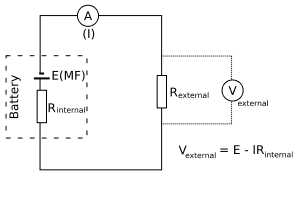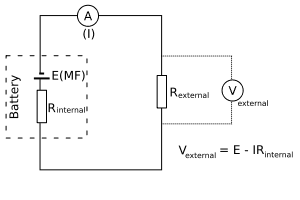# Circuits .. what is this question asking?

## Homework Statement

A battery has an emf E and internal resistance r. A variable resistor R is connected across the terminals of the battery. Find the values of R such that

(a) the potential difference across the terminals is a maximum;
(b) the current in the circuit is a maximum;
(c) the power delivered to the resistor is a maximum.

I=E/(R+r)

## The Attempt at a Solution

Im not sure if question (a) is talking about max voltage we can run on the circuit, or is just talking about the EMF in which case it never changes.

b) I assume when R=0 we get max current, no resistant means max current.
C) Max Power transfer theorem says when R=r we get max power.

Any help with a) greatly appreciated.

nsaspook
The question asks about the terminal voltage (the external connection points to the battery) in question (a).•Fat_Squirrel
The question asks about the terminal voltage (the external connection points to the battery) in question (a).Cheers.

Ok, in that case its when the current through the circuit is zero, so basically when we have a switch or a resistor with infinite resistance?

That makes sense.
I assume I'm okay on the other two?

nsaspook
That makes sense.
I assume I'm okay on the other two?

Yes, for perfect circuits and batteries.

•Fat_Squirrel
Thanks heaps. Feeling stupid. Not good when I'm stuck on one of the first questions. Appreciate the quick response. Now I can enjoy my wine in peace.

nsaspook